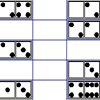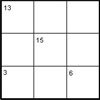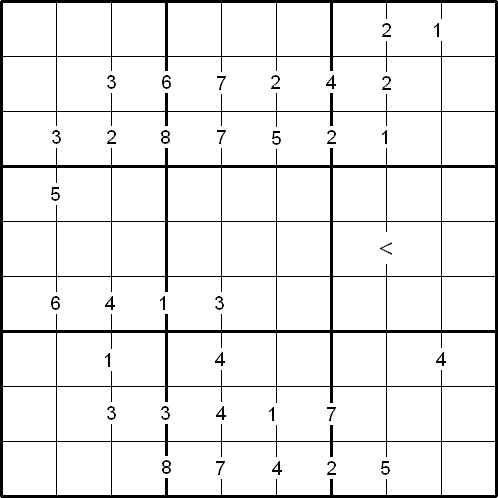#### You may also like### Prime Magic

Place the numbers 1, 2, 3,..., 9 one on each square of a 3 by 3 grid so that all the rows and columns add up to a prime number. How many different solutions can you find?### Domino Magic Rectangle

An ordinary set of dominoes can be laid out as a 7 by 4 magic rectangle in which all the spots in all the columns add to 24, while those in the rows add to 42. Try it! Now try the magic square...### Intersection Sums Sudoku

A Sudoku with clues given as sums of entries.

# Difference Sudoku

##### Age 14 to 16 Challenge Level:

By Henry Kwok#### Rules of Difference Sudoku

Like the standard Sudoku, the object of the puzzle is to fill the whole $9 \times 9$ grid with numbers from $1$ to $9$, so that each row, each column, and each of the nine $3 \times 3$ squares contain all nine digits.

There are special clue-numbers placed on the border lines between selected pairs of adjacent squares of the grid. Each clue-number is the difference between the two numbers in the adjacent squares.

For example, a clue-number $7$ on the border line between two adjacent squares means that the possible pairs of numbers for the squares on either side must be one of the following combinations: $1$ and $8$, $8$ and $1$, $2$ and $9$, or $9$ and $2$.

The inequality sign indicates that the number in row 5 column 7 is smaller than the number in row 5 column 8.

As this variant has only one inequality sign on the border line, it is called Minimal Difference Sudoku.

A printable version of the problem can be found here.